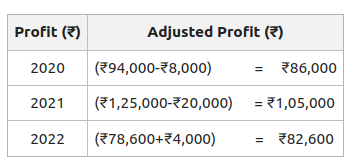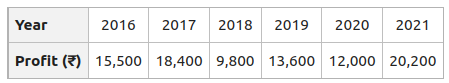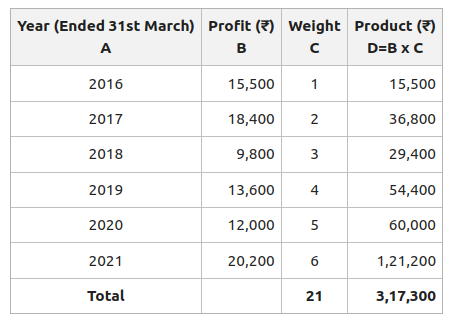Open in App
Not now

# Average Profit Method of calculating Goodwill

• Difficulty Level : Basic
• Last Updated : 22 Aug, 2022

Goodwill can be defined as the value of the business. It is the intangible asset of the business. An enterprise earns more profit than the normal profit because of Goodwill. Goodwill can be defined as the reputation of the business earned through hard work, honesty, quality, and customer satisfactory services. Goodwill helps to earn profits in the future without much effort because of the retention of the customers. Thus, because of the existence of goodwill, one firm earns more profit than the other firms in the same industry. It is an attractive force that brings old customers to that old place. Goodwill is the value of the share of profit sacrificed by the sacrificing partner, in partnership.

“Goodwill is the probability that the old customers will resort to the old place.” – Lord Eldon

Meaning of Average Profit Method:

Average means to divide among a number, according to a given proportion. It is the set of values computed by dividing the sum of the values by their numbers. Under the Average Profit Method, goodwill can be calculated by two methods:

#### A. Simple Average Profit Method:

In the Simple Average Profit Method, normal profits are earned by the business for a specified number of years. Profits earned are totalled and their average is determined. To calculate goodwill, average profit is multiplied by the number of years’ purchases. The number of years’ purchase means for how many years the firm will earn the same amount of profit because of its past efforts after the change of ownership. When a running business is purchased, the purchaser has to pay an amount for goodwill for being in the position to earn profit in the initial years of business.

Steps of Average Profit Method of Calculating Goodwill:

Step 1: Calculate the normal past business profit for each year by deducting abnormal gains and non-business expenses.

Step 2: Add the profits calculated for the past given years.

Step 3: Calculate the Average Profit (or Average Maintainable Profit) as:

Average Profit =Step 4: Calculate the goodwill by applying the formula:

Goodwill = Average Profit X Number of years of purchase

#### B. Weighted Average Profit Method:

In the Weight Average Profit Method, each profit is multiplied by the number of assigned weights, i.e. 1,2,3,4.. to ascertain the value of goodwill. Weighted Average Profit is calculated by dividing the total products of profits by the total weights, and the goodwill is ascertained by multiplying the weighted average profit by the agreed number of years’ purchase.

Steps of Weighted Average Profit Method of Calculating Goodwill:

Step 1: Assigning weights to the profits. The highest weight is assigned to the profit of the most recent year.

Step 2: The product of profits is calculated by multiplying the weight with the respective profit of that particular year.

Step 3: Weighted Average Profit is then calculated by dividing the Total of products of profits by the Total Weights.

Weighted Average Profit =Step 4: The value of Goodwill is calculated by multiplying the Weighted Average Profit by the Number of years of purchase.

Goodwill = Weighted Average Profit × Number of years of purchase

Illustration 1:

Calculate the value of goodwill of the firm of three years’ purchase from the previous five-year profits earned:

Solution

Average Profit ==== ₹12,820

Goodwill = Average profit x Number of years of purchase
= ₹12,800 x 3
= ₹38,460

Illustration 2:

Calculate the value of goods on two years’ purchase of average profit of the firm from the following:

2020: ₹94,000 (excluding ₹8,000 as insurance premium on the firm’s property-now to be insured)

2021: ₹1,25,000 (including the abnormal gain of ₹20,000)

2022: ₹78,600 (after charging an abnormal loss of ₹4,000)

Solution:Total Profits for the last 3 years = ₹86,000 + ₹1,05,000 + ₹82,600
= ₹2,73,600

Average Profit == ₹91,200

Goodwill = Average profit x Number of years of purchase
= ₹91,200 x 2
= ₹1,82,400

Illustration 3:

Calculate the value of Goodwill based on four years’ purchase of the weighted average profit after assigning weights 1,2,3,4,5 and 6, respectively to the profits for 2016, 2017, 2018, 2019, 2020, and 2021. The profits of the firm for the year ended 31st March for the last six years were:Solution:Weighted Average Profit === ₹15,110

Goodwill = Weighted Average profit x Number of years of purchase

= ₹15,110 x 4
= ₹60,440

My Personal Notes arrow_drop_up
Related Articles